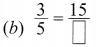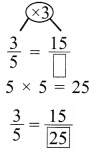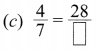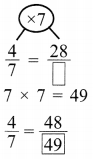# DAV Class 4 Maths Chapter 9 Brain Teasers Solutions

The DAV Class 4 Maths Solutions and DAV Class 4 Maths Chapter 9 Brain Teasers Solutions of Fractions offer comprehensive answers to textbook questions.

## DAV Class 4 Maths Ch 9 Brain Teasers Solutions

Question 1.
(a) Which of the following is a proper fraction?
(i) $$\frac{17}{19}$$
(ii) $$\frac{15}{14}$$
(iii) $$\frac{13}{9}$$
(iv) $$\frac{18}{17}$$
(i) $$\frac{17}{19}$$

(b) Fraction whose numerator is greater than the denominator is called ___________ fraction.
(i) Unit
(ii) Proper
(iii) Improper
(iv) Mixed
(iii) Improper

(c) 7$$\frac{1}{5}$$ written as an improper fraction is ___________
(i) $$\frac{5}{36}$$
(ii) $$\frac{36}{5}$$
(iii) $$\frac{71}{5}$$
(iv) $$\frac{5}{71}$$
(ii) $$\frac{36}{5}$$

(d) Tick (✓) the missing numerator in(i) 10
(ii) 18
(iii) 8
(iv) 38
(ii) 18

(e) Which of the following is not equivalent to $$\frac{3}{7}$$?
(i) $$\frac{6}{14}$$
(ii) $$\frac{30}{70}$$
(iii) $$\frac{12}{20}$$
(iv) $$\frac{15}{35}$$
(iii) $$\frac{12}{20}$$Question 2.
Fill in the blanks.
(a) $$\frac{5}{6}$$ is a ___________ fraction.
Proper

(b) 3$$\frac{1}{7}$$ is a ___________ number.
Mixed.

(c) $$\frac{19}{7}$$ is an ___________ fraction.
Improper

(d) $$\frac{1}{8}, \frac{1}{3}$$ and $$\frac{1}{16}$$ are ___________ fractions.
Unit

(e) Fractions whose denominators are same are called ___________ fractions.
Like

Question 3.
Write the next three equivalent fractions.
(a) $$\frac{2}{6}, \frac{4}{12}, \frac{6}{18}$$, ___________, ___________, ___________ , ___________
2 × 4 = 8 The next no. = $$\frac{8}{24}$$
6 × 4 = 24
Then $$\frac{5}{40}, \frac{6}{48}$$
$$\frac{8}{24}, \frac{10}{30}, \frac{12}{36}$$

(b) $$\frac{1}{8}, \frac{2}{16}, \frac{3}{24}$$, ___________, ___________, ___________ , ___________
1 × 4 = 4
8 × 4 = 32
The next no. = $$\frac{4}{32}$$
Then $$\frac{5}{40}, \frac{6}{48}$$
$$\frac{4}{32}, \frac{5}{40}, \frac{6}{48}$$

Question 4.
Find the missing numerator or denominator.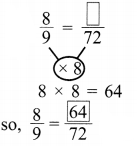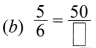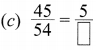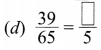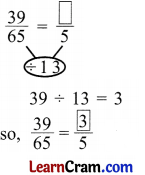Question 5.
Complete the pattern:
(a) $$\frac{1}{4}, \frac{1}{16}, \frac{1}{64}$$, ___________, ___________
$$\frac{1}{256}, \frac{1}{1024}$$

(b) 1, $$\frac{2}{3}, \frac{4}{9}, \frac{8}{27}$$, ___________, ___________
$$\frac{16}{81}, \frac{32}{243}$$

Question 1.
For each of following figure write the fraction.(a) $$\frac{4}{12}$$
(b) $$\frac{3}{8}$$
(c) $$\frac{6}{12}$$

Question 2.
Find equivalent fraction.
(a) $$\frac{2}{3}, \frac{12}{18}$$2 × 18 = 36
3 × 12 = 36
So, $$\frac{2}{3}=\frac{12}{18}$$

(b) $$\frac{5}{3}, \frac{15}{9}$$5 × 9 = 45
3 × 15 = 45
So, $$\frac{5}{3}=\frac{15}{9}$$

(c) $$\frac{2}{5}, \frac{10}{20}$$2 × 20 = 40
5 × 10 = 50
So, $$\frac{2}{5} \neq \frac{10}{20}$$

(d) $$\frac{3}{7}, \frac{12}{35}$$3 × 35 = 75
7 × 12 = 84
So, $$\frac{3}{7} \neq \frac{12}{35}$$Question 3.
Name each pair of the fraction.
(i) $$\frac{2}{15}, \frac{7}{15}$$
Like fraction, proper fraction.

(ii) $$\frac{1}{3}, \frac{2}{5}$$
Unlike fraction, proper fraction.

(iii) $$\frac{1}{7}, \frac{1}{5}$$
Unit fraction.

(iv) $$\frac{9}{6}, \frac{8}{3}$$
Improper fraction, unlike fraction

(v) 3$$\frac{1}{2}$$, 4$$\frac{1}{5}$$
Mixed number.

(vi) $$\frac{3}{5}, \frac{1}{3}$$
Unlike fraction, proper fraction.

Question 4.
Change the following into mixed number.
(a) $$\frac{56}{12}$$
$$\frac{56}{12}$$
= 56 ÷ 12
= 4$$\frac{8}{12}$$(b) $$\frac{67}{11}$$
$$\frac{67}{11}$$
= 67 ÷ 11
= 6$$\frac{1}{11}$$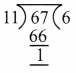(c) $$\frac{73}{9}$$
$$\frac{73}{9}$$
= 73 ÷ 9
= 8$$\frac{1}{9}$$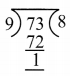(d) $$\frac{54}{7}$$
$$\frac{54}{7}$$
= 54 ÷ 7
= 7$$\frac{5}{7}$$Question 5.
Find the missing numerator or denominator.